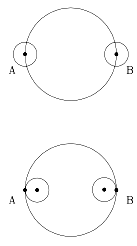# (8c) The Moon's Distance--1Index5c. Coordinates6. The Calendar6a. Jewish Calendar7.Precession8. The Round Earth8a. The Horizon8b. Parallax8c. Moon dist. (1)8d. Moon dist. (2)9a. Earth orbits Sun?9b. The Planets9c. Copernicus
to Galileo10. Kepler's Laws10a. Scale of Solar Sys.11a. Ellipses
and First Law

The Greek scholar Aristarchus wrote around 270 BC a book "On the Sizes and Distances" (of the Sun and Moon).

He calculated their distances in units of the Earth's radius, viewing, Earth Sun and Moon as a spheres (the Earth's radius was later estimated by Erathostenes). In particular, he estimated the distance of the Sun.

All his values were much smaller than the ones later derived by astronomers and used now. According to the Wikipedia article cited above, a calculation by his method, using values he proposed, gives (Re=Earth radius, Rm=Moon radius)

 Dimension Aristarchus Modern Sun's radius in Re 6.7 109 Earth's radius in Rm 2.85 350 Earth-Moon distance in Re 20 60.32 Earh-Sun distance in Re 380 23500

The inaccuracies can perhaps be traced by noting that Aristarchus, combining his estimates, took the (nearly equal) apparent sizes of the Moon and Sun in the sky as 2 degrees, about 4 times too large, and claimed the angle Moon-Sun-Earth, at a time when exactly half the Moon was in sunlight, equaled about 3 degrees (much too big).

A century later Hipparchus found an independent method based on a solar eclipse, which according to the wikipedia article placed the Moon 67 Re away (a rough calculation here gives 90 Re.)

 Actually, one can also derive the Moon's distance from the duration of totality in a lunar eclipse, as some later astronomers did.       Totality in a central lunar eclipse lasts 109 minutes, and this may be taken as the the time taken for the Moon to cross the Earth's shadow, roughly equal to the Earth's diameter 2Re. The orbital period of the Moon is about 27 days or 38880 minutes, the time it takes for the Moon to return to a position on the celestial sphere. The time from one "new moon" to the next is 2.21 days longer, because it requires the Moon's position to catch up with that ot the Sun, and the Sun's position in the sky changes during the time, due of the Earth's orbital motion).       If the Moon orbits at constant speed in a circle of radius R, its lenght is 2πR, where the Greek π is about 3.14 (more accurately, 3.1415926...), then 2πR/2Re = 38880/107 = 363.36... R/Re ~ 115 while the true value is about 60. However the time the center of the Moon needs to pass the total width of the Earth shadow is longer than totality. In the figure shown below totality lasts while the Moon covers AB in the bottom figure, whereas the crossing the width of the shadow it needs cover AB in the top one, with the Moon partially eclipsed near A and B.Also, the Earth's shadow has only approximately the width of 2r. It would have very nearly a width of 2r if the Sun were a point-like light source (exactly that width if it were infinitely far away). Actually, however, the Sun is large enough to appear as a disk which covers about half a degree of the sky. As a result, the Earth's shadow is not a cylinder but a gradually narrowing cone, and at the Moon's distance it is already about 25% narrower than 2r. The tip of that cone is not far from the Lagrangian L2 point, near the planned position of the Webb space telescope (see end of section #34a further along).       Aristarchus has also been credited (by Archimedes) as the first scholar to claim that the Earth moved around the Sun, not the other way around. He apparently had not yet put the Sun at the center when he wrote "On the Sizes and Distances." That "heliocentric" interpretation was not accepted by astronomers until the age of Copernicus and Galileo, nearly 2000 years later. Questions from Users: ***   How do we know Earth is bigger than the Moon?       *** Aristarchus Teachers using this web page will find a related lesson plan at Lhipprc2.htm   It belongs to a set of lesson plans whose home page is at Lintro.htm. Further Exploring:    #8d  How distant is the Moon?--2 Author and Curator:   Dr. David P. Stern      Mail to Dr.Stern:   stargaze("at" symbol)phy6.org .

Last updated: 30 August 2017